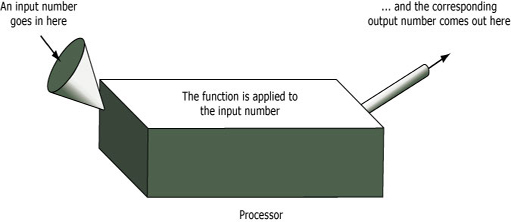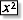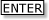Tapping into mathematics

Start this free course now. Just create an account and sign in. Enrol and complete the course for a free statement of participation or digital badge if available.

Free course

# 7 Mathematical functions

## 7.1 Introduction

As you have probably observed, there are many mathematical functions on your calculator, but most users only need to use a few of them regularly. This is an opportunity to be introduced to some of the more useful ones informally. Many functions are directly visible on the keys of the keyboard, but your calculator may have additional functions, e.g. on a MATH menu.

This section is designed to give you a whistle-stop introduction to some of the important functions on your calculator, but you are not expected to understand all the mathematical functions. Rather the aim is to give you an overview, which you will be able to build upon later.

The best way to explore a new calculator button is to try it out using very simple numbers. Some examples are included to get you started. Note down any insights you uncover about what these keys do. Also, do not forget to practise saying the names of the function – it is important to be able to talk mathematics as well as write it.

You may have noticed some mathematical jargon in the preceding pages; terms like the ‘square’ of a number and an ‘exponent’. Learning mathematical language is an important part of learning mathematics. The word ‘function’, in particular, is a much-used word in mathematics and it is worth thinking about for a few moments before moving on.

One way of thinking about a mathematical function is to imagine a processor with an input and an output, perhaps rather like the one shown in Figure 2.Figure 2 A function represented as an input–output machine

A number is supplied to the input of the processor. Inside the processor it is converted to another number (do not worry for the moment about how this is done), and this new number is then delivered to the output. The function itself is the relationship between the input number and the output number.

In a sense, this is how the calculator operates. When a number is entered, it is displayed on the calculator's screen and treated as an input. You then select the particular processor you want by pressing the appropriate keys.

Pressing, say, 7instructs the calculator to work out the square of the input, 7, and to display the result, 49, the output, on the screen.

MU120_4MCAL